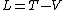# Lagrangian Function

## Lagrangian function

A function of the generalized coordinates and velocities of a dynamical system from which the equations of motion in Lagrange's form can be derived. The Lagrangian function is denoted by L(q1, …, qf; &dot;q1, …, &dot;qf; t). For systems in which the forces are derivable from a potential energy V, if the kinetic energy is T, the equation below holds.See Lagrange's equations

McGraw-Hill Concise Encyclopedia of Physics. © 2002 by The McGraw-Hill Companies, Inc.
The following article is from The Great Soviet Encyclopedia (1979). It might be outdated or ideologically biased.

## Lagrangian Function

(or kinetic potential), expressed in terms of generalized coordinates qi, generalized velocities coordinates gi, generalized velocities i, and time t. In the simplest case of a conservative system, the Lagrangian function is equal to the difference between the kinetic energy T and the potential energy Π of the system expressed in terms of qi and qi, that is, L = T(qi, q̇i, t) —Π (q̇i). If we know the Lagrangian function, it is possible to construct differential equations of motion of a mechanical system using the principle of least action.

The Great Soviet Encyclopedia, 3rd Edition (1970-1979). © 2010 The Gale Group, Inc. All rights reserved.

## Lagrangian function

[lə′grän·jē·ən ‚fəŋk·shən]
(mechanics)
The function which measures the difference between the kinetic and potential energy of a dynamical system.
McGraw-Hill Dictionary of Scientific & Technical Terms, 6E, Copyright © 2003 by The McGraw-Hill Companies, Inc.
References in periodicals archive ?
Then the Lagrangian function is constructed based on the theory of Lagrangian multiplier method and can be expressed as
The problem is converted to minimize the Lagrangian function
We add an adaptive quadratic term to the quadratic model of the augmented Lagrangian function. In each iteration, we solve a simple unconstrained subproblem to obtain the trail step.
Then the Lagrangian function that one minimizes is structured as the following form:
As for the unknown parameters x [member of] Rn and [theta] [member of] Rm, the previous system yields a solution by evaluating the Hessian matrix of the Lagrangian function, which is a rather difficult method.
The objective function is then transformed into Lagrangian function. The major aim of the study is to estimate the cost parameters.
For this purpose, we make use of Lagrangian function / and maximize revenue, given the constant costs C, pY(K, L).
The Lagrangian function of F(q)with respect to the transmission power([P.sup.(0).sub.k,i], [P.sup.(1).sub.k,i]) for given sensing time [??] is derived as:
First, the system of equations is written as a Lagrangian function where [[lambda].sub.0], [[lambda].sub.1], and [eta] are Lagrangian multipliers.
Step 2 : Then construct the Lagrangian function L(x, q, r, = Where x = (x1, x2,....xn), r = (r1, r2, ...rn),.
In equation (1) L is the Lagrangian function, which depends on the coordinates and velocities and sometimes also on the time.

Site: Follow: Share:
Open / Close